# Thermal Resistance

Thermal resistance (Rth) is an important concept and index to heat transfer calculation and product development, Rth is the ratio of temperature difference to thermal power between two points. The greater the thermal resistance, the worse the heat transfer performance; conversely, the smaller the thermal resistance, the better the heat transfer.

There are 3 heat transfer mechanisms: heat conduction, heat convection and heat radiation.
The thermal resistance of heat conduction is determined by material property and the geometric dimensions.Equation of heat convection is obtained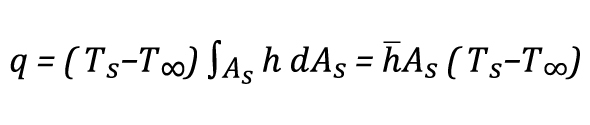Thus, thermal resistance of heat convection is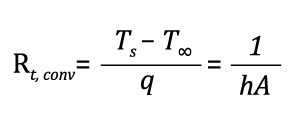Where h varies with moving fluid conditions, the faster the flow, the higher the convection  coefficient h.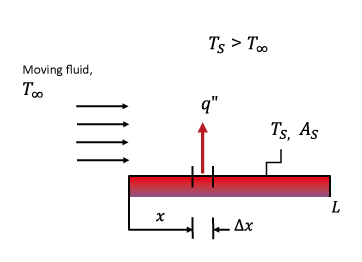Thermal resistance from a heat sink base to the ambient (Rth or Rb-a) is used to describe it’s thermal performance, and the unit is K/W or °C/W

• Rth = (Tb-Ta)/Pd
• Rth or Rb-a: Thermal resistance
• Pd: Thermal power
• Tb: Heat sink base temperature
• Ta: Ambient temperature

The following figure is an example for heat sink Rth calculation, heat source’s heating power is Pd.
the temperature at bottom of heat sink is Tb and the ambient temperature is T amb;
Thermal resistance Rth of the heat sink is (Tb-T amb)/Pd
The Rth contains conduction thermal resistance and convection thermal resistance as previously stated.
To improve overall thermal performance, we may use other materials with higher thermal conductivity, or add in heat pipes to effectively drop conductional thermal resistance.
Or we may optimize the fin and air flow design to reduce convective thermal resistance.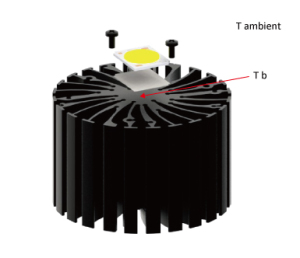A Mechatronix example is listed for LED luminaire heatsink thermal performance calculation and analysis,

As an example we take a LED COB model, which has a nominal forward current If of 450mA and a maximal forward current of 900mA.
We will drive the module at a forward current of 500mA with a forward voltage Vf of 35.5V.
The maximal case temperature Tc is 105°C but in our design we aim at a life time case temperature of 75°C.
The ambient temperature for our application is 35°C. The electrical power Pe = Vf x If or 35.5V x 0.5A = 17.75W.
The dissipated power Pd = Pe x efficiency where the efficiency of the COB is around 32% or 17.75W x 0.68 = 12.07W.
This is the amount of energy which need to be cooled down.
dT is the temperature difference between the case temperature Tc we want to acquire and the ambient temperature Ta
dT = Tc = Ta or 75°C – 35°C = 40°C
The required maximal thermal resistance Rth of the LED cooler + the thermal interface material Rth = dT / Pd = 40°C / 12.07W = 3.31°C/W
The thermal interface you use has a major impact on the performance.
We recommend to use either arctic silver, a good thermal grease or a thin 0.1 to 0.15mm phase change or graphite thermal pad.
In this case the thermal resistance from the interface material will be between 0.1 and 0.2°C/W.
This interface resistance you substract from the calculated interface resistance to determine the cooling performance your heat sink needs to be.
So Rth heatsink = 3.31°C/W – 0.2°C/W = 3.11°C/W maximal.
Any led cooler which does better than a thermal resistance (lower value) of 3.11°C/W in free air conditions would make that our LED case temperature Tc will remain below the required 75°C.
Keep in mind that an enclosure around the LED cooler, tilting and other variations will affect the performance of the LED cooler.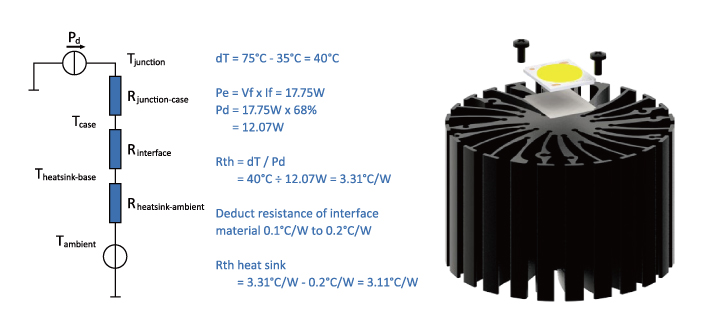Founded in August 2010, Cooling House is an established Asia-based design and manufacturing company for innovative thermal solutions and is headquartered in Taipei. Cooling House holds patents for several cooling technologies and product concepts.